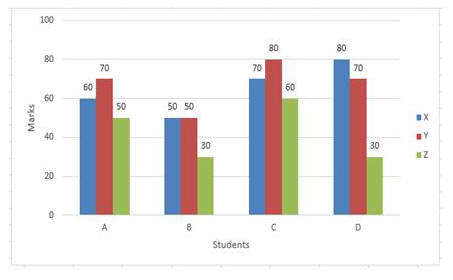# Important SSC CHSL Maths Questions: Solve Here

In this blog, we will be sharing with you the Important SSC CHSL Maths Questions, solve the questions in the minimum time with accuracy.

You can solve different types of questions here, as we have covered each and every type that can be asked in the upcoming SSC CHSL 2019-20 examination.

Want to Practice what toppers’ are practicing? Practice Here

## Important SSC CHSL Maths Questions: Solve Here

Q) With reference to a number greater than one, the difference between itself and its reciprocal is 25% of the sum of itself and its reciprocal. By how much percentage (correct one decimal place) is the fourth power of the number greater than its square?

A. 62.5
B. 66.7
C. 64.5
D. 57.8

Q) The full marks for a paper is 300. The break-up of the marks into theory (X), practical (Y) and (Z) project, which are the three components of evaluation is 6 : 5 : 4. In order to pass one has to score at least 40%, 50% and 50% respectively in XYZ and 60% in aggregate. The marks scored by four students ABC and D are shown in the given Bar Graph.How much percentage marks more than B has C scored in practical?

A. 40
B. 30
C. 20
D. 60

Topper took 20 seconds. Can you beat the time? Test Here

Q) AB and CD are two chords of a circle which intersect at a point O inside the circle. It is given that, AB = 10 cm, CO= 1.5 cm and DO = 12.5 cm. What is the ratio between the larger and smaller among AO and BO?

A. 3:1
B. 4:1
C. 7:3
D.  3:2

Q) During a practice session in a stadium an athlete runs along a circular track and her performance is observed by her coach standing at a point on the circle and also by her physiotherapist standing at the centre of the circle. The coach finds that she covers an angle of 72° in 1 min. What will be the angle covered by her in 1 second according to the measurement made by her physiotherapist?

A. 1.2
B. 4.8
C. 2.4
D. It depends on the position of the coach on the circular track

Check your all India standing with a free

Q) The value of
18.43 x 18.43 – 6.57 x 6.57 / 11.86 is:

A. 25
B. 23.62
C. 26
D. 24.12

Q) A purchased two articles for Rs.200 and Rs.300 respectively and sold at gains of 5% and 10% respectively. What was his overall gain percentage?

A. 5
B. 8
C. 9
D. 6

Want To practice more SSC CHSL Maths questions? Take a complete quiz here and test your preparations.

Q) The ten digit number 2y600000z8 is exactly divisible by 24. If y ≠ 0 and z ≠ 0, then the least value of (y + z) is equal to:

A. 2
B. 9
C. 8
D. 5

Q) The average of 1088 real numbers is zero. At most how many of them can be negative?

A. 1087
B. 88
C.100
D. 544

Know where you stand. Attempt a free mock tests and analyze your performance ⇒ Test Yourself Here

Q) The two diagonals of a rhombus are respectively, 14 cm and 48 cm. The perimeter of the rhombus is equal to:

A. 160 cm
B. 100 cm
C. 180 cm
D. 80 cm

Q) A certain sum was invested on simple interest. The amount to which it had grown in five years was 5/4 times the amount to which it had grown in three years. The percentage rate of interest was:

A. 20%
B. 15%
C. 10%
D. 25%

Solve More questions and test your Maths section preparation for SSC CHSL 2019-20. among your peers.

Q)  The ration of the square of a number to the reciprocal of its cube is 243/16807. What is the number?

A. 2/7
B. 3/7
C. 5/7
D. 7/3

Q) O, G, I and H are respectively the circumcentre, centroid, incentre and orthocentre of an equilateral triangle. Which of these points are identical?

A. O and I only
B. O, G, I and H
C. O and G only
D. O, G and H

Toppers’ took the SSC CHSL Maths Questions mock tests and found them the best. Want to beat the toppers’ score and analyze your performance?⇒

Q) What is the value of cosec² 30° + sin² 45° + sec² 60° +tan² 30°?

A. 53/6
B. 8
C. 9
D.25/3

Q)  For 0° ≤ θ ≤ 90°, what is θ, when √3cosec θ + sin θ = 1?

A. 90º
B. 30º
C. 45º
D. 60º

Take a free mock test and analyze your performance

Q) An article having marked price, Rs.900, was sold for Rs.648 after two successive discounts. The first discount was 20%. What was the percentage rate of the second discount?

A. 10
B. 5
C. 15
D. 12.5

Q) If 10/7 (1 – 2.43 X 10¯3) = 1.417 + x, then x is equal to:

A. 0.0081
B. 0.417
C. 0.81
D. 0.411

Check your all India standing with a free

Q) The simplified value of {5/4 of (7/3 ÷ 7/5) – 17/12} + 1/9 ÷ 7/3 + 2/7 + 1/6 is :

A. 1
B. 7/6
C. 7/3
D. 3/2

Q)  If (3x + 1)³ + (x – 3)³ + (4 – 2x)³ + 6 (3x + 1) (x – 3) (x – 2) = 0, then x is equal to:

A. -1
B. 1
C. 1/2
D. -1/2

Want to attempt more SSC CHSL Maths Questions? Solve Here & Analyze Your Performance

Q) The platform of a station 400 m long starts exactly where the last span of a bridge 1.2 km long ends. How long will a train 200 m long and traveling at the speed of 72 km/h take to cover the distance between the starting point of the span of the bridge and the far end of the platform?

A. 1.2 min
B. 1.3 min
C. 1.5 min
D. 1.6 min